# ePSproc - basic plotting development, XC version

03/07/20, simplified version to look at XC data and betas only.

Aims

## Setup

:

# Standard libs
import sys
import os
from pathlib import Path
import numpy as np
import xarray as xr

from datetime import datetime as dt
timeString = dt.now()

# For reporting
import scooby
# scooby.Report(additional=['holoviews', 'hvplot', 'xarray', 'matplotlib', 'bokeh'])
# TODO: set up function for this, see https://github.com/banesullivan/scooby

:

# Installed package version
# import epsproc as ep

# ePSproc test codebase (local)
if sys.platform == "win32":
modPath = r'D:\code\github\ePSproc'  # Win test machine
else:
modPath = r'/home/femtolab/github/ePSproc/'  # Linux test machine

sys.path.append(modPath)
import epsproc as ep

* plotly not found, plotly plots not available.

:

# Plotting libs
# Optional - set seaborn for plot styling
import seaborn as sns
sns.set_context("paper")  # "paper", "talk", "poster", sets relative scale of elements
# https://seaborn.pydata.org/tutorial/aesthetics.html
# sns.set(rc={'figure.figsize':(11.7,8.27)})  # Set figure size explicitly (inch)
# https://stackoverflow.com/questions/31594549/how-do-i-change-the-figure-size-for-a-seaborn-plot
# Wraps Matplotlib rcParams, https://matplotlib.org/tutorials/introductory/customizing.html
sns.set(rc={'figure.dpi':(120)})

from matplotlib import pyplot as plt  # For addtional plotting functionality
# import bokeh

import holoviews as hv
from holoviews import opts


:

# Load data from modPath\data
dataPath = os.path.join(modPath, 'data', 'photoionization')
dataFile = os.path.join(dataPath, 'n2_3sg_0.1-50.1eV_A2.inp.out')  # Set for sample N2 data for testing

# Scan data file
dataXS = ep.readMatEle(fileIn = dataFile, recordType = 'CrossSection')  # XS info currently not set in NO2 sample file.

*** ePSproc readMatEle(): scanning files for DumpIdy segments.

*** Scanning file(s)
['/home/femtolab/github/ePSproc/data/photoionization/n2_3sg_0.1-50.1eV_A2.inp.out']

*** Reading ePS output file:  /home/femtolab/github/ePSproc/data/photoionization/n2_3sg_0.1-50.1eV_A2.inp.out
Expecting 2 symmetries.
Scanning CrossSection segments.
Expecting 102 DumpIdy segments.
Found 102 dumpIdy segments (sets of matrix elements).

Processing segments to Xarrays...
Processed 102 sets of DumpIdy file segments, (0 blank)
*** ePSproc readMatEle(): scanning files for CrossSection segments.

*** Scanning file(s)
['/home/femtolab/github/ePSproc/data/photoionization/n2_3sg_0.1-50.1eV_A2.inp.out']

*** Reading ePS output file:  /home/femtolab/github/ePSproc/data/photoionization/n2_3sg_0.1-50.1eV_A2.inp.out
Expecting 2 symmetries.
Scanning CrossSection segments.
Expecting 3 CrossSection segments.
Found 3 CrossSection segments (sets of results).
Processed 3 sets of CrossSection file segments, (0 blank)


## Xarray plotting

• Xarray wraps Matplotlib functionality. (And can be modified using Matplotlib calls, and will pick up Seaborn styling if set.)

• Easy to use, supports line and surface plots, with faceting.

• Doesn’t support high dimensionality directly, need to subselect and/or facet and then pass set of 1D or 2D values.

• Not interactive in Jupyter Notebook, or HTML, output.

:

# Plot with faceting on symmetry
daPlot = ep.matEleSelector(dataXS, thres=1e-2, dims = 'Eke', sq = True).squeeze()
daPlot.plot.line(x='Eke', col='Sym', row='Type');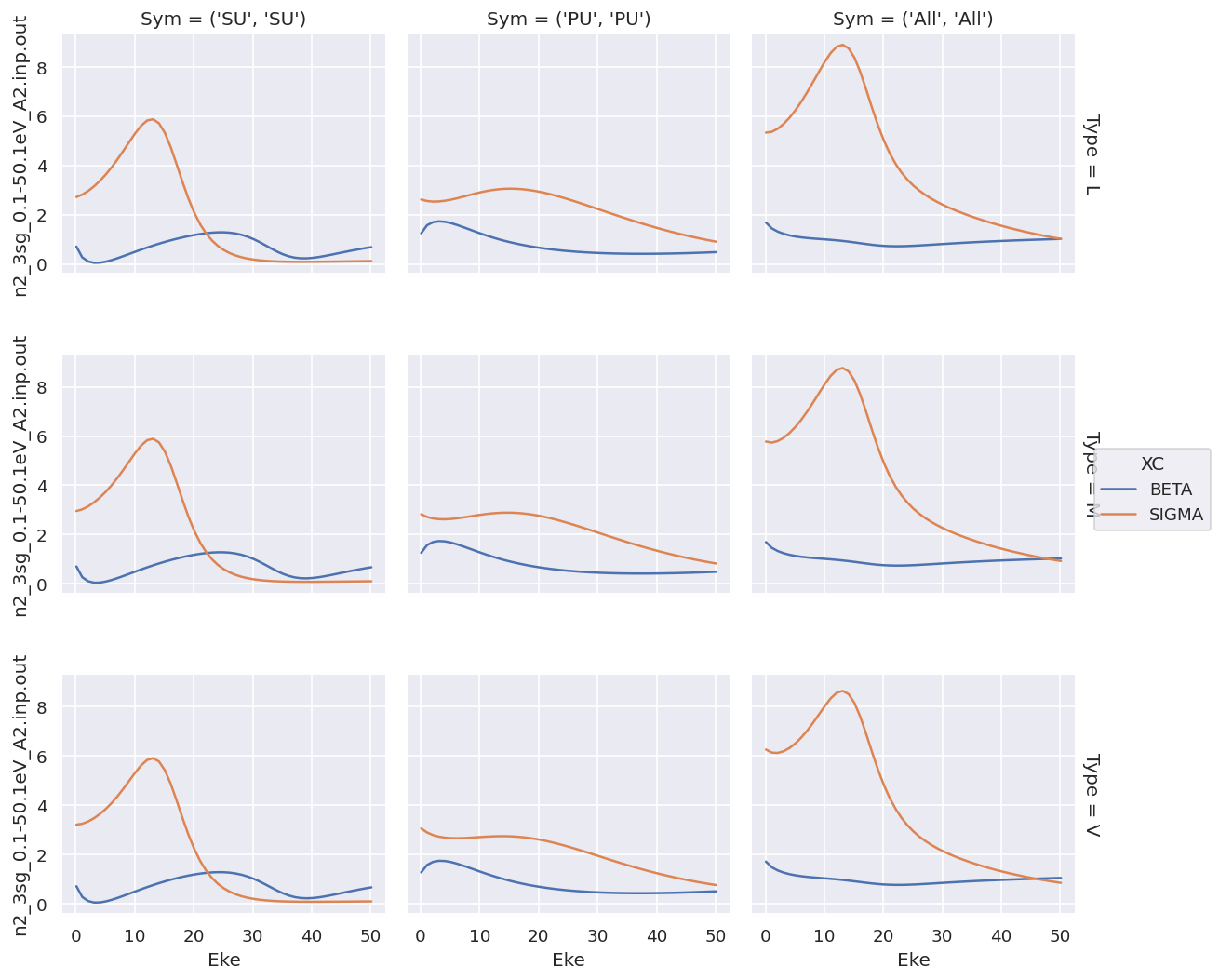For XC data this provides a complete overview, but the shared y-axis is not ideal for observing the details of the $$\beta$$ parameters.

Plotting with faceting by type is similar…

:

# For XS data - this works nicely, except (1) no control over ordering, (2) same y-axis for all data types.
# Plot with faceting
daPlot = ep.matEleSelector(dataXS, thres=1e-2, dims = 'Eke', sq = True).squeeze()
# daPlot.pipe(np.abs).plot.line(x='Eke', col='Type', row='XC');
daPlot.plot.line(x='Eke', col='Type', row='XC');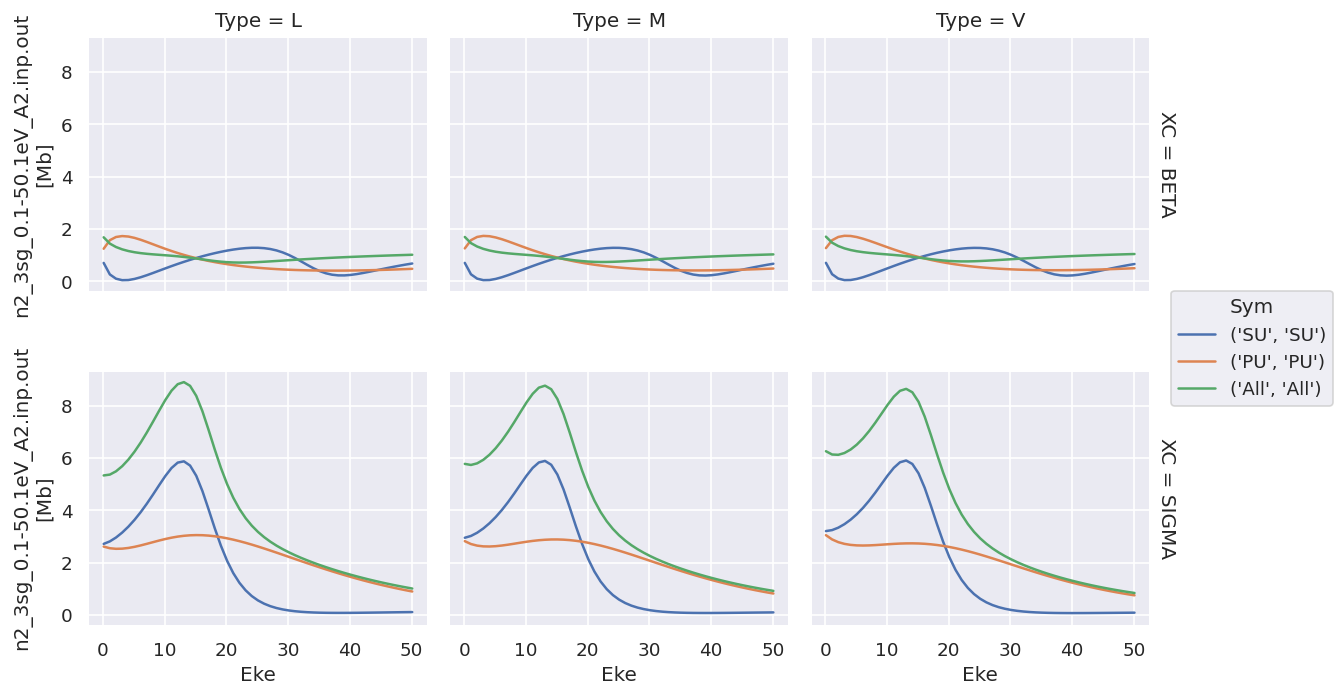Plotting values independently solves the issue…

:

# Try plotting independently... this allows for independent y-axis scaling over data types.
daPlot.sel({'XC':'SIGMA'}).plot.line(x='Eke', col='Type');
daPlot.sel({'XC':'BETA'}).plot.line(x='Eke', col='Type');
# OK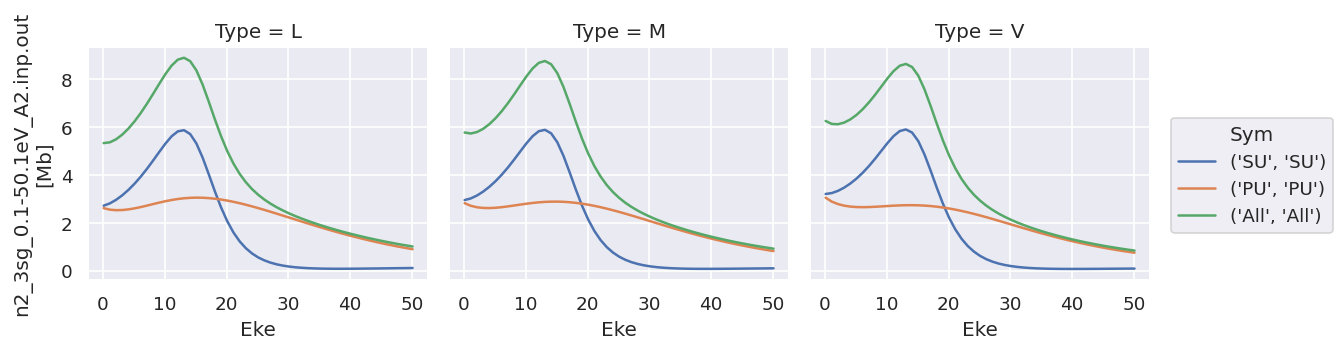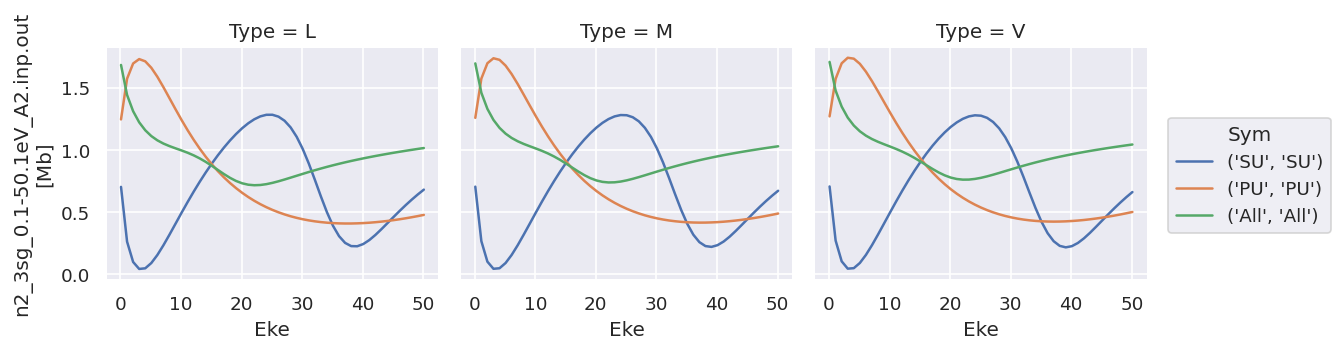As does converting the data structure to an Xarray Dataset (rather than Dataarray, which is assumed to hold homogeneous data), see below for more details.

### Data reformat & datasets

Main issue with plotting as above is different datatypes (ranges), and also ways to extend to multiple datasets.

:

# Default formatting from ep.readMatEle() is stacked Xarray, with XC as a dimension
dataXS.coords

:

Coordinates:
* Type     (Type) object 'L' 'M' 'V'
Ehv      (Eke) float64 15.68 16.68 17.68 18.68 ... 62.68 63.68 64.68 65.68
* XC       (XC) object 'BETA' 'SIGMA'
* Sym      (Sym) MultiIndex
- Total    (Sym) object 'SU' 'PU' 'All'
- Cont     (Sym) object 'SU' 'PU' 'All'
* Eke      (Eke) float64 0.1 1.1 2.1 3.1 4.1 5.1 ... 46.1 47.1 48.1 49.1 50.1

:

# Test: stack to dataset with XC dim removed.
# This should be correct for keeping datatypes consistent.
# Can then add an additional dim for multiple orbitals, theory vs. expt, etc.
ds = xr.Dataset({'sigma':dataXS.sel({'XC':'SIGMA'}).drop('XC'),
'beta':dataXS.sel({'XC':'BETA'}).drop('XC')})
ds

:

<xarray.Dataset>
Dimensions:  (Eke: 51, Sym: 3, Type: 3)
Coordinates:
* Type     (Type) object 'L' 'M' 'V'
Ehv      (Eke) float64 15.68 16.68 17.68 18.68 ... 62.68 63.68 64.68 65.68
* Sym      (Sym) MultiIndex
- Total    (Sym) object 'SU' 'PU' 'All'
- Cont     (Sym) object 'SU' 'PU' 'All'
* Eke      (Eke) float64 0.1 1.1 2.1 3.1 4.1 5.1 ... 46.1 47.1 48.1 49.1 50.1
Data variables:
sigma    (Sym, Eke, Type) float64 2.719 2.954 3.209 ... 1.013 0.9229 0.8423
beta     (Sym, Eke, Type) float64 0.7019 0.7036 0.7053 ... 1.014 1.028 1.042

:

# In this case there's not much direct plotting available, but variables can be called independently as above.
ds['sigma'].plot.line(x='Eke', col='Type');
ds['beta'].plot.line(x='Eke', col='Type');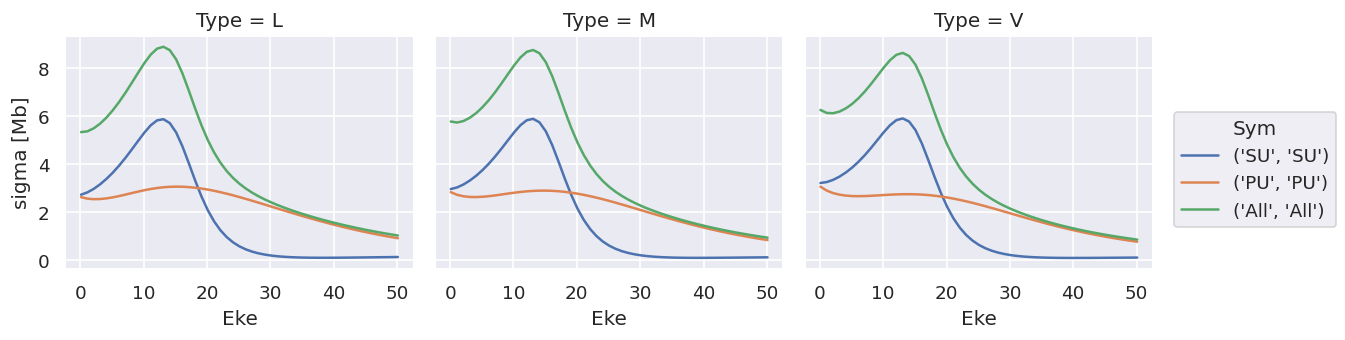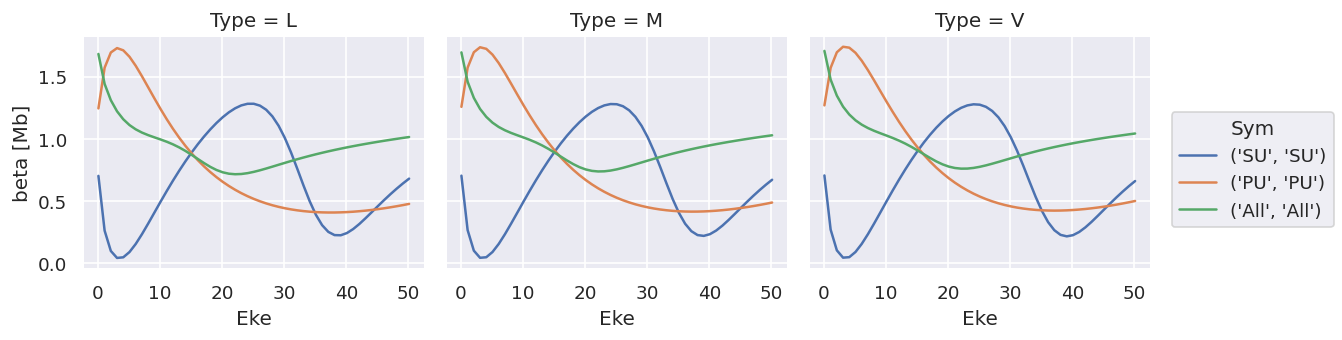Note that the units are (incorrectly) labelled as the same for both, this should be fixed!

TODO: change file IO to treat sigma & beta independently!

## ep.lmPlot

Designed for multi-dim plotting of matrix elements or $$\beta$$ parameters, see plotting routines page for details.

## Holoviews

Requires conversion from Xarray data-array or dataset, but then pretty flexible.

Basics here from HV tabular datasets and Gridded Datasets intro pages, plus embelishments.

Issues:

• Doesn’t handle multi-indexing in data? Seem to have to unstack() before plotting, but TBD.

• Unlinking y-axes currently not working in data-array case, not sure why. Tried a few methods (cell magic, or setting various things in opts - see test notebook for more). Using datasets gets around this however.

### With Matplotlib backend

:

# Init - without this no plots will be displayed
hv.extension('matplotlib'):

# hv_ds = hv.Dataset(dataSet.sel({'Type':'L', 'it':1, 'Cont':'SU'}).squeeze().unstack('LM').sel({'m':0,'mu':0}).real)  # OK
# hv_ds = hv.Dataset(dataSet.sel({'Type':'L', 'it':1, 'Cont':'SU'}).squeeze().unstack('LM').real)  # OK
hv_ds = hv.Dataset(dataXS.sel({'Type':'L'}).unstack(['Sym']).sum(['Total'])) # OK - reduce Sym dims.
# hv_ds = hv.Dataset(dataXS.sel({'Type':'L'}).unstack(['Sym']))
print(hv_ds)

:Dataset   [XC,Eke,Cont]   (n2_3sg_0.1-50.1eV_A2.inp.out)


Basic plotting will generate plots of specified type, with specified key dimensions, plus sliders or lists for other dims.

Note, as before, that the data is here contained in a single ND array.

:

matEplot = hv_ds.to(hv.Curve, kdims=["Eke"])
matEplot.opts(aspect=1)

:

:

# Basic layout functionality for gridding
matEplot = hv_ds.to(hv.Curve, kdims=["Eke"])
matEplot.layout().cols(3)

# matEplot.select(Cont={'SU','PU'}).layout().cols(2)  # Can also use select here to set a subset of plots

: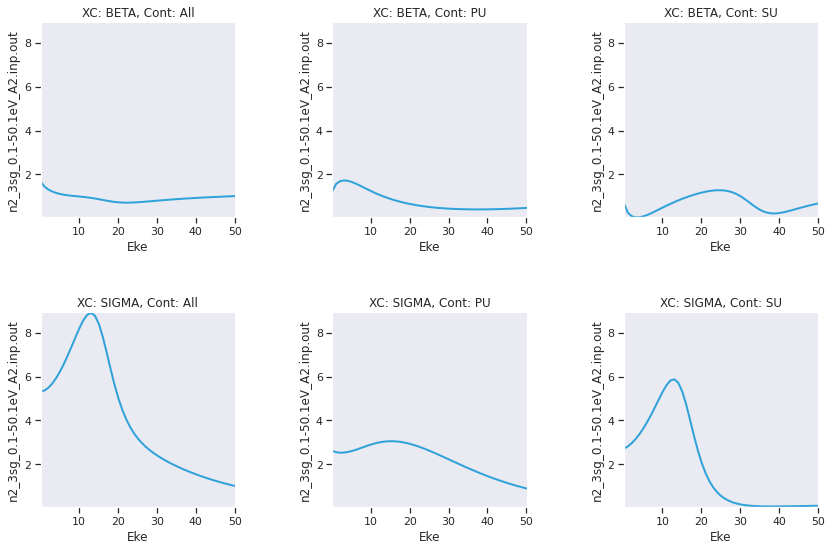Generally this isn’t so interesting, since it provides essentially the same functionality as the Xarray.plot() methods, albeit with a little more control.

### With bokeh backend

Using HV with Bokeh on the backend is nice, since it provides interactivity in Notebook + HTML output.

:

# Load extension
hv.extension('bokeh')